# Guide to R and Python in a Single Jupyter NotebookWhy pick one when you can use both at the same time?

The war between R and Python users has been raging for several years. With most of the old school statisticians being trained on R and most computer science and data science departments in universities instead preferring Python, both have pros and cons. The main cons I have noticed in practice are in the packages that are available for each language.

As of 2019, the R packages for cluster analysis and splines are superior to the Python packages of the same kind. In this article, I will show you, with coded examples, how to take R functions and datasets and import and utilize then within a Python-based Jupyter notebook.

• Importing (base) R functions
• Importing R library functions
• Populating vectors R understands
• Populating dataframes R understands
• Populating formulas R understands
• Running models in R
• Getting results back to Python
• Getting model predictions in R
• Plotting in R

Linear/Polynomial Regression

Firstly, we will look at performing basic linear and polynomial regression using imported R functions. We will examine a dataset looking at diabetes with information about C-peptide concentrations and acidity variables. Do not worry about the contents of the model, this is a commonly used example in the field of generalized additive models, which we will look at later in the article.

diab = pd.read_csv("data/diabetes.csv")print("""
# Variables are:
#  subject:  subject ID number
#  age:      age diagnosed with diabetes
#  acidity:  a measure of acidity called base deficit
#  y:        natural log of serum C-peptide concentration
#
# Original source is Sockett et al. (1987)
# mentioned in Hastie and Tibshirani's book
display(diab.dtypes)
display(diab.describe())


We can then plot the data:

ax0 = diab.plot.scatter(x='age',y='y',c='Red',title="Diabetes data") #plotting direclty from pandas!
ax0.set_xlabel("Age at Diagnosis")
ax0.set_ylabel("Log C-Peptide Concentration");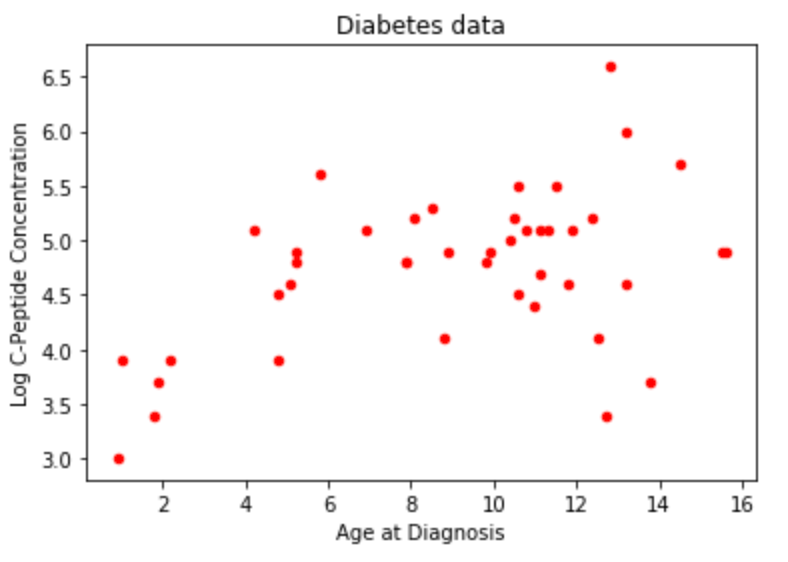Linear regression with statsmodel. You may need to install the package in order to follow the code, you can do this with pip install statsmodel.

• In Python, we work from a vector of target values and a design matrix we built ourself (e.g. from PolynomialFeatures).
• Now, statsmodel’s formula interface can help build the target value and design matrix for you.
#Using statsmodels
import statsmodels.formula.api as sm

model1 = sm.ols('y ~ age',data=diab)
fit1_lm = model1.fit()


Now we build a data frame to predict values on (sometimes this is just the test or validation set)

• Very useful for making pretty plots of the model predictions — predict for TONS of values, not just whatever’s in the training set
x_pred = np.linspace(0,16,100)
predict_df = pd.DataFrame(data={"age":x_pred})


Use get_prediction(<data>).summary_frame() to get the model's prediction (and error bars!)

prediction_output = fit1_lm.get_prediction(predict_df).summary_frame()


Plot the model and error bars

ax1 = diab.plot.scatter(x='age',y='y',c='Red',title="Diabetes data with least-squares linear fit")
ax1.set_xlabel("Age at Diagnosis")
ax1.set_ylabel("Log C-Peptide Concentration")
ax1.plot(predict_df.age, prediction_output['mean'],color="green")
ax1.plot(predict_df.age, prediction_output['mean_ci_lower'], color="blue",linestyle="dashed")
ax1.plot(predict_df.age, prediction_output['mean_ci_upper'], color="blue",linestyle="dashed");
ax1.plot(predict_df.age, prediction_output['obs_ci_lower'], color="skyblue",linestyle="dashed")
ax1.plot(predict_df.age, prediction_output['obs_ci_upper'], color="skyblue",linestyle="dashed");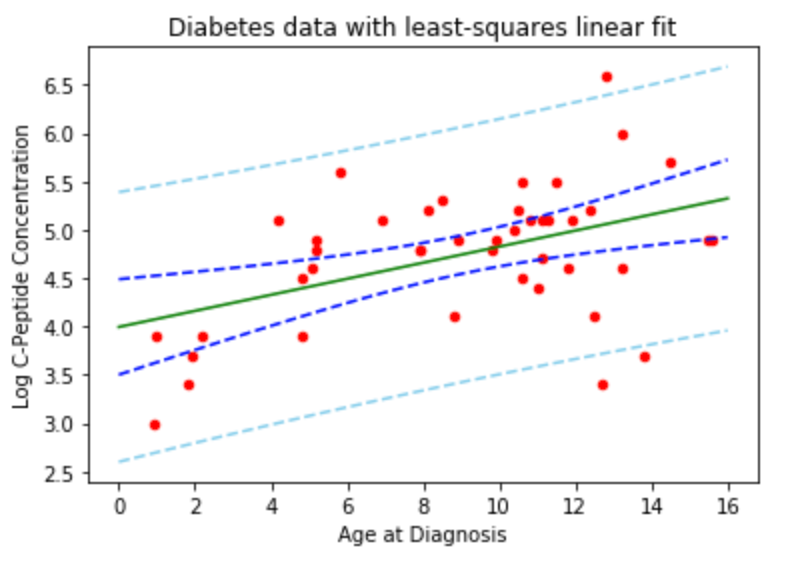We can also fit a 3rd-degree polynomial model and plot the model error bars in two ways:

• Route1: Build a design df with a column for each of age, age2, age3
fit2_lm = sm.ols(formula="y ~ age + np.power(age, 2) + np.power(age, 3)",data=diab).fit()
poly_predictions = fit2_lm.get_prediction(predict_df).summary_frame()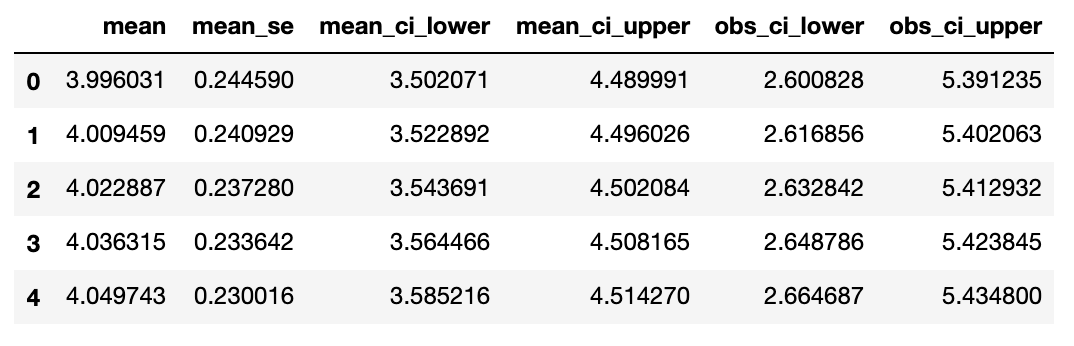• Route2: Just edit the formula
ax2 = diab.plot.scatter(x='age',y='y',c='Red',title="Diabetes data with least-squares cubic fit")
ax2.set_xlabel("Age at Diagnosis")
ax2.set_ylabel("Log C-Peptide Concentration")
ax2.plot(predict_df.age, poly_predictions['mean'],color="green")
ax2.plot(predict_df.age, poly_predictions['mean_ci_lower'], color="blue",linestyle="dashed")
ax2.plot(predict_df.age, poly_predictions['mean_ci_upper'], color="blue",linestyle="dashed");
ax2.plot(predict_df.age, poly_predictions['obs_ci_lower'], color="skyblue",linestyle="dashed")
ax2.plot(predict_df.age, poly_predictions['obs_ci_upper'], color="skyblue",linestyle="dashed");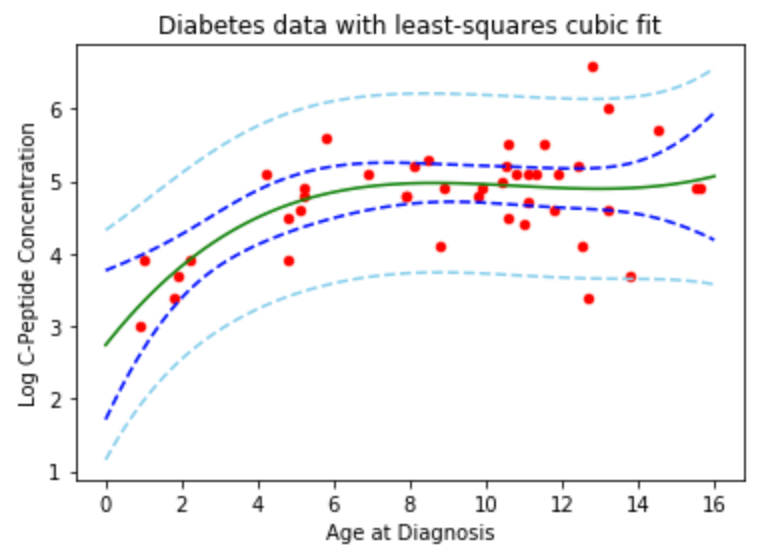This did not use any features of the R programming language. Now, we can repeat the analysis using functions from R.

Linear/Polynomial Regression, but make it R

After this section, we’ll know everything we need to in order to work with R models. The rest of the lab is just applying these concepts to run particular models. This section, therefore, is your ‘cheat sheet’ for working in R.

What we need to know:

• Importing (base) R functions
• Importing R Library functions
• Populating vectors R understands
• Populating DataFrames R understands
• Populating Formulas R understands
• Running models in R
• Getting results back to Python
• Getting model predictions in R
• Plotting in R

Importing R functions

To import R functions we need the rpy2 package. Depending on your environment, you may also need to specify the path to the R home directory. I have given an example below for how to specify this.

# if you're on JupyterHub you may need to specify the path to R
#import os
#os.environ['R_HOME'] = "/usr/share/anaconda3/lib/R"
import rpy2.robjects as robjects


To specify an R function, simply use robjects.r followed by the name of the package in square brackets as a string. To prevent confusion, I like to use r_ for functions, libraries, and other objects imported from R

r_lm = robjects.r["lm"]
r_predict = robjects.r["predict"]
#r_plot = robjects.r["plot"] # more on plotting later
#lm() and predict() are two of the most common functions we'll use


Importing R libraries

We can import individual functions, but we can also import entire libraries too. To import an entire library, you can extract the importr package from rpy2.robjects.packages .

from rpy2.robjects.packages import importr
#r_cluster = importr('cluster')
#r_cluster.pam;


Populating vectors R understands

To specify a float vector that can interface with Python packages, we can use the robjects.FloatVector function. The argument to this function references the data array that you wish to convert to an R object, in our case, the age and y variables from our diabetes dataset.

r_y = robjects.FloatVector(diab['y'])
r_age = robjects.FloatVector(diab['age'])
What happens if we pass the wrong type?
How does r_age display?
How does r_age print?


Populating Dataframes R understands

We can specify individual vectors, and we can also specify entire dataframes. This is done by using the robjects.DataFrame function. The argument to this function is a dictionary specifying the name and the vector (obtained from robjects.FloatVector ) associated with the name.

diab_r = robjects.DataFrame({"y":r_y, "age":r_age})
How does diab_r display?
How does diab_r print?


Populating formulas R understands

To specify a formula, for example, for regression, we can use the robjects.Formula function. This follows the R syntax dependent variable ~ independent variables . In our case, the output y is modeled as a function of the age variable.

simple_formula = robjects.Formula("yage")
simple_formula.environment["y"] = r_y #populate the formula's .environment, so it knows what 'y' and 'age' refer to
simple_formula.environment["age"] = r_age


Notice in the above formula we had to specify the FloatVector’s associated with each of the variables in our formula. We have to do this as the formula does not automatically relate our variable names to variables that we have previously specified —  they have not yet been associated with the robjects.Formula object.

Running Models in R

To specify a model, in this case a linear regression model using our previously imported r_lm function, we need to pass our formula variable as an argument (this will not work unless we pass an R formula object).

diab_lm = r_lm(formula=simple_formula) # the formula object is storing all the needed variables


Instead of specifying each of the individual float vectors related to the robjects.Formula object, we can reference the dataset in the formula itself (as long as this has been made into an R object itself).

simple_formula = robjects.Formula("yage") # reset the formula
diab_lm = r_lm(formula=simple_formula, data=diab_r) #can also use a 'dumb' formula and pass a dataframe


Getting results back to Python

Using R functions and libraries is great, but we can also analyze our results and get them back to Python for further processing. To look at the output:

diab_lm #the result is already 'in' python, but it's a special object


We can also check the names in our output:

print(diab_lm.names) # view all names


To take the first element of our output:

diab_lm #grab the first element


To take the coefficients:

diab_lm.rx2("coefficients") #use rx2 to get elements by name!


To put the coefficients in a Numpy array:

np.array(diab_lm.rx2("coefficients")) #r vectors can be converted to numpy (but rarely needed)


Getting Predictions

To get predictions using our R model, we can create a prediction dataframe and use the r_predict function, similar to how it is done using Python.

# make a df to predict on (might just be the validation or test dataframe)
predict_df = robjects.DataFrame({"age": robjects.FloatVector(np.linspace(0,16,100))})# call R's predict() function, passing the model and the data
predictions = r_predict(diab_lm, predict_df)


We can use the rx2 function to extract the ‘age’ values:

x_vals = predict_df.rx2("age")


We can also plot our data using Python:

ax = diab.plot.scatter(x='age',y='y',c='Red',title="Diabetes data")
ax.set_xlabel("Age at Diagnosis")
ax.set_ylabel("Log C-Peptide Concentration");ax.plot(x_vals,predictions); #plt still works with r vectors as input!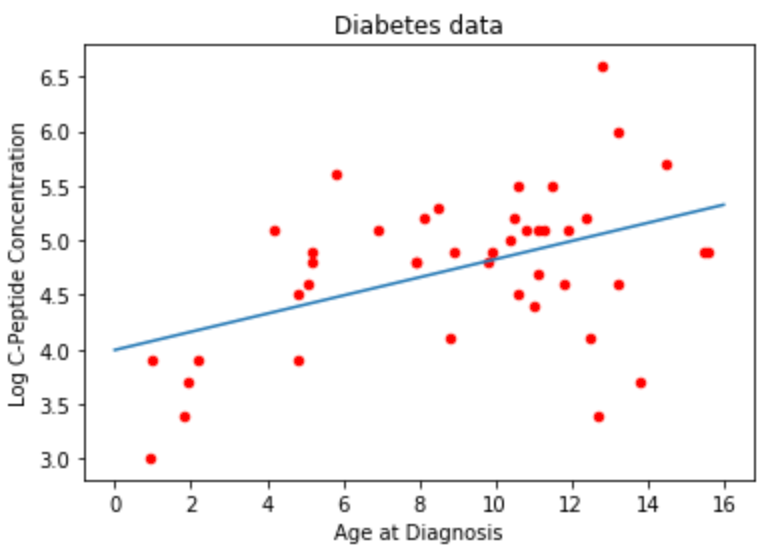We can also plot using R, although this is slightly more involved.

Plotting in R

To plot in R, we need to turn on the %R magic function using the following command:

%load_ext rpy2.ipython

• The above turns on the %R “magic”.
• R’s plot() command responds differently based on what you hand to it; different models get different plots!
• For any specific model search for plot.modelname. For example, for a GAM model, search plot.gam for any details of plotting a GAM model.
• The %R “magic” runs R code in ‘notebook’ mode, so figures display nicely
• Ahead of the plot(<model>) code we pass in the variables R needs to know about (-i is for "input")
%R -i diab_lm plot(diab_lm);


The documentation for the lm() function is here, and a prettier version (same content) is here. When Googling, prefer rdocumentation.org when possible. Sections:

• Usage: gives the function signature, including all optional arguments
• Arguments: What each function input controls
• Details: additional info on what the function does and how arguments interact. Often the right place to start reading
• Value: the structure of the object returned by the function
• References: The relevant academic papers

Example

As an example to test our newly acquired knowledge, we will try the following:

• Add confidence intervals calculated in R to the linear regression plot above. Use the interval= argument to r_predict()(documentation here). You will have to work with a matrix returned by R.
• Fit a 5th-degree polynomial to the diabetes data in R. Search the web for an easier method than writing out a formula with all 5 polynomial terms.

Confidence intervals:

CI_matrix = np.array(r_predict(diab_lm, predict_df, interval="confidence"))
ax = diab.plot.scatter(x='age',y='y',c='Red',title="Diabetes data")
ax.set_xlabel("Age at Diagnosis")
ax.set_ylabel("Log C-Peptide Concentration");
ax.plot(x_vals,CI_matrix[:,0], label="prediction")
ax.plot(x_vals,CI_matrix[:,1], label="95% CI", c='g')
ax.plot(x_vals,CI_matrix[:,2], label="95% CI", c='g')
plt.legend();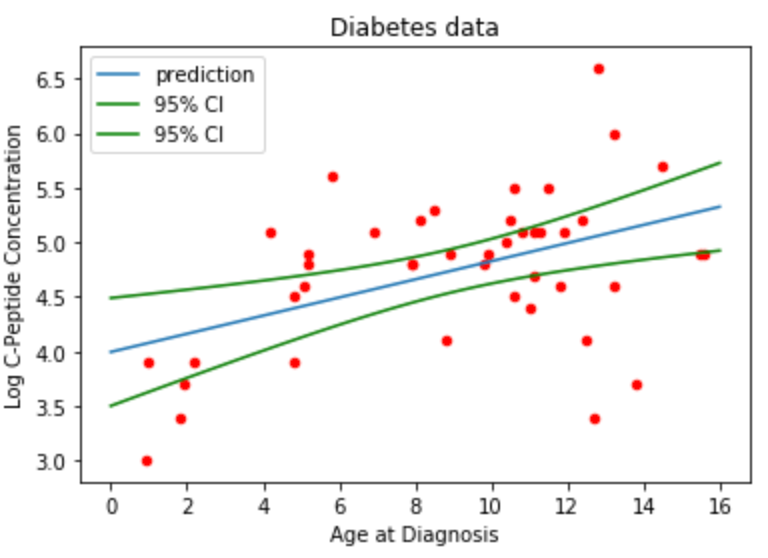5-th degree polynomial:

ploy5_formula = robjects.Formula("y~poly(age,5)") # reset the formula
diab5_lm = r_lm(formula=ploy5_formula, data=diab_r) #can also use a 'dumb' formula and pass a dataframe
predictions = r_predict(diab5_lm, predict_df, interval="confidence")
ax = diab.plot.scatter(x='age',y='y',c='Red',title="Diabetes data")
ax.set_xlabel("Age at Diagnosis")
ax.set_ylabel("Log C-Peptide Concentration");
ax.plot(x_vals,predictions);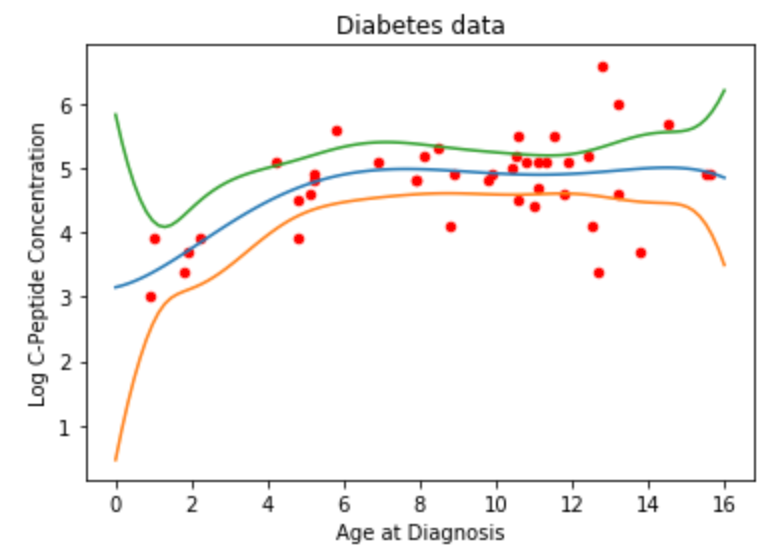Lowess Smoothing

Now that we know how to use R objects and functions within Python, we can look at cases that we might want to do this. The first we will example is Lowess smoothing.

Lowess smoothing is implemented in both Python and R. We’ll use it as another example as we transition languages.

Python

In Python, we use the statsmodel.nonparametric.smoothers_lowess to perform lowess smoothing.

from statsmodels.nonparametric.smoothers_lowess import lowess as lowessss1 = lowess(diab['y'],diab['age'],frac=0.15)
ss2 = lowess(diab['y'],diab['age'],frac=0.25)
ss3 = lowess(diab['y'],diab['age'],frac=0.7)
ss4 = lowess(diab['y'],diab['age'],frac=1)ss1[:10,:] # we get back simple a smoothed y value for each x value in the data


Notice the clean code to plot different models. We’ll see even cleaner code in a minute.

for cur_model, cur_frac in zip([ss1,ss2,ss3,ss4],[0.15,0.25,0.7,1]):   ax = diab.plot.scatter(x='age',y='y',c='Red',title="Lowess Fit, Fraction = {}".format(cur_frac))
ax.set_xlabel("Age at Diagnosis")
ax.set_ylabel("Log C-Peptide Concentration")
ax.plot(cur_model[:,0],cur_model[:,1],color="blue")

plt.show()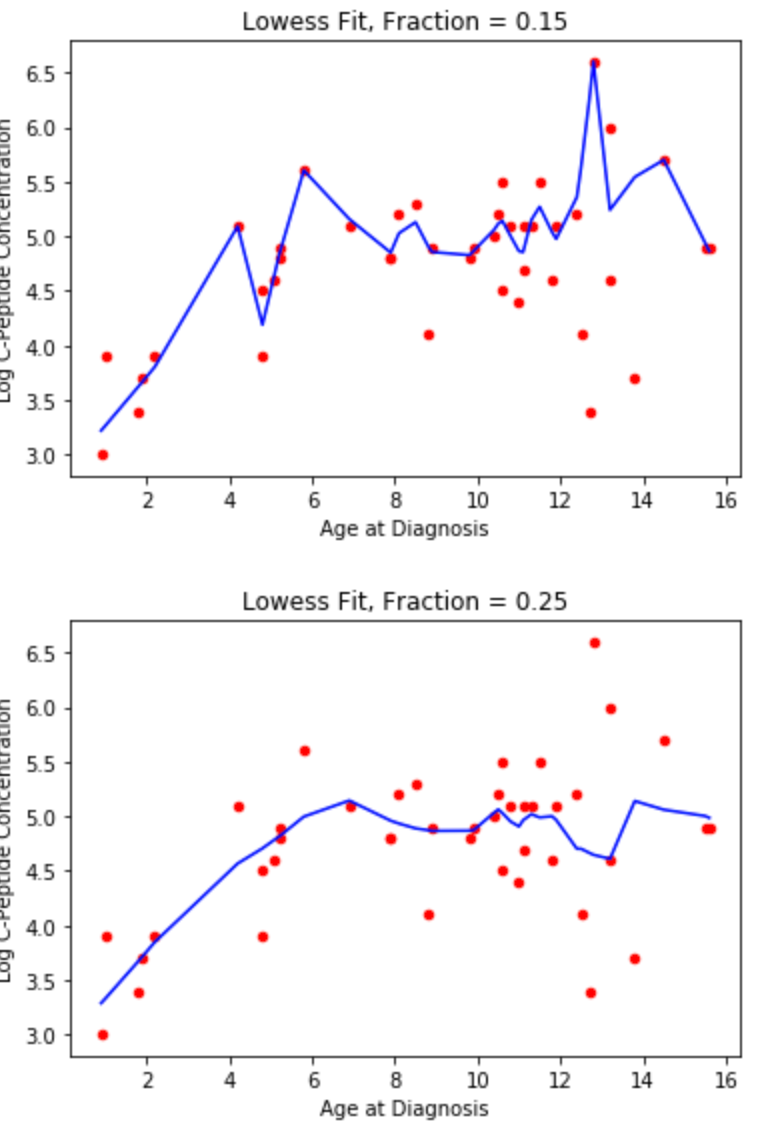R

To implement Lowess smoothing in R we need to:

• Import the loess function.
• Send the data over to R.
• Call the function and get results.
r_loess = robjects.r['loess.smooth'] #extract R function
r_y = robjects.FloatVector(diab['y'])
r_age = robjects.FloatVector(diab['age'])ss1_r = r_loess(r_age,r_y, span=0.15, degree=1)ss1_r #again, a smoothed y value for each x value in the data


Varying span

Next, some extremely clean code to fit and plot models with various parameter settings. (Though the zip() method seen earlier is great when e.g. the label and the parameter differ)

for cur_frac in [0.15,0.25,0.7,1]:

cur_smooth = r_loess(r_age,r_y, span=cur_frac)   ax = diab.plot.scatter(x='age',y='y',c='Red',title="Lowess Fit, Fraction = {}".format(cur_frac))
ax.set_xlabel("Age at Diagnosis")
ax.set_ylabel("Log C-Peptide Concentration")
ax.plot(cur_smooth, cur_smooth, color="blue")

plt.show()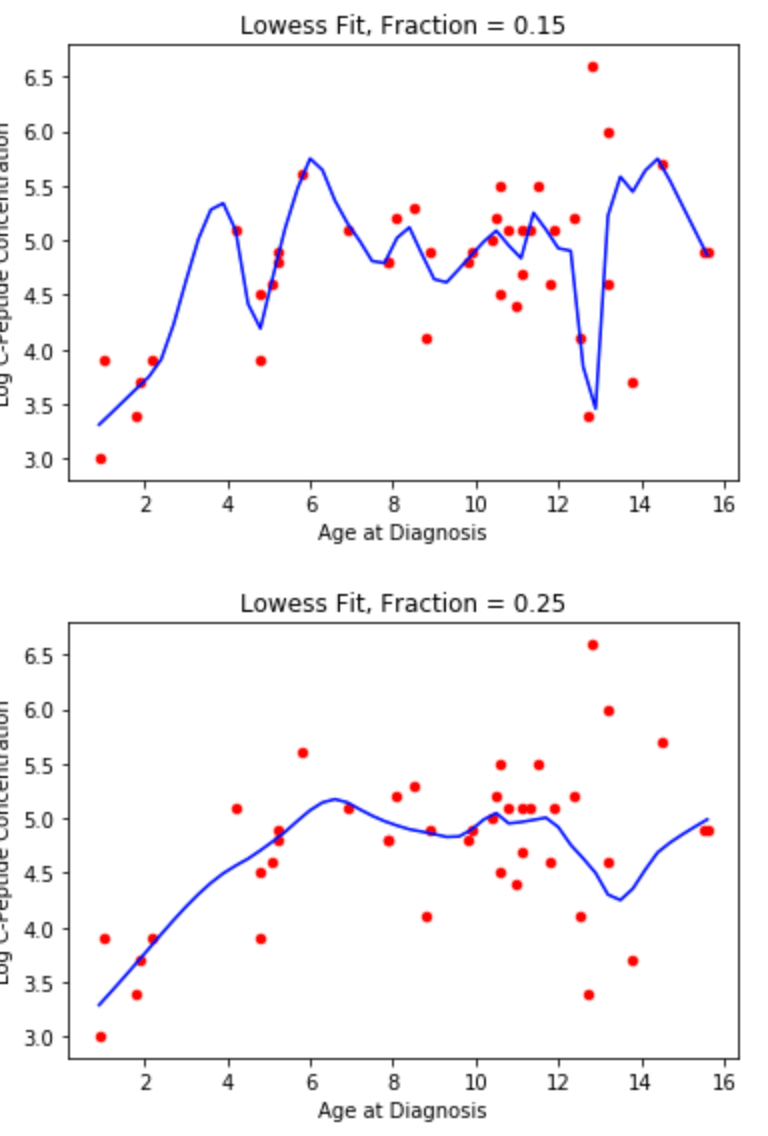The next example we will look at is smoothing splines, these models are not well supported in Python and so using R functions is preferred.

Smoothing Splines

From this point forward, we’re working with R functions; these models aren’t (well) supported in Python.

For clarity: this is the fancy spline model that minimizes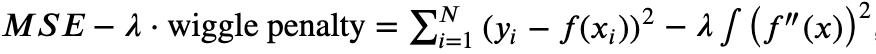across all possible functions f. The winner will always be a continuous, cubic polynomial with a knot at each data point.

Some things to think about are:

• Any idea why the winner is cubic?
• How interpretable is this model?
• What are the tunable parameters?

To implement the smoothing spline, we only need two lines.

r_smooth_spline = robjects.r['smooth.spline'] #extract R function# run smoothing function
spline1 = r_smooth_spline(r_age, r_y, spar=0)


Smoothing Spline Cross-Validation

R’s smooth_spline function has a built-in cross validation to find a good value for lambda. See package docs.

spline_cv = r_smooth_spline(r_age, r_y, cv=True) lambda_cv = spline_cv.rx2("lambda")ax19 = diab.plot.scatter(x='age',y='y',c='Red',title="smoothing spline with $\lambda=$"+str(np.round(lambda_cv,4))+", chosen by cross-validation")
ax19.set_xlabel("Age at Diagnosis")
ax19.set_ylabel("Log C-Peptide Concentration")
ax19.plot(spline_cv.rx2("x"),spline_cv.rx2("y"),color="darkgreen")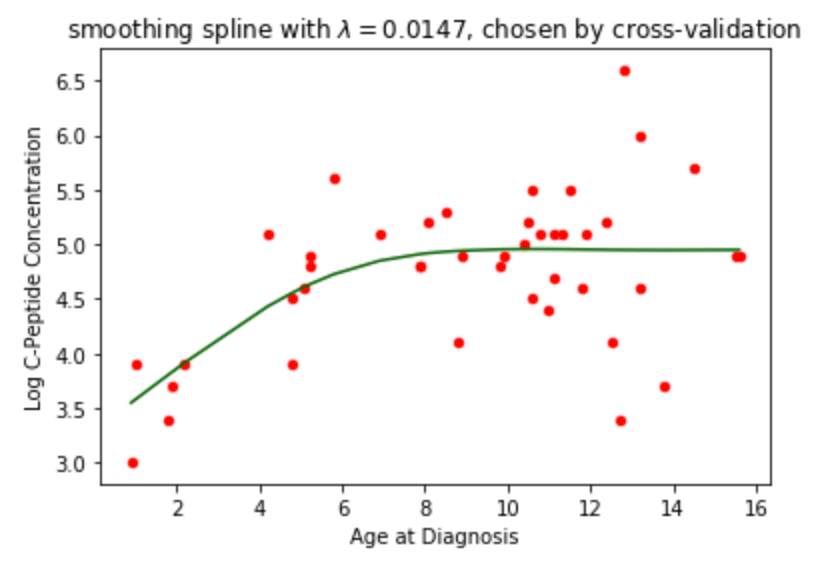Natural & Basis Splines

Here, we take a step backward on model complexity, but a step forward in coding complexity. We’ll be working with R’s formula interface again, so we will need to populate Formulas and Dataframes.

Some more food for thought:

• In what way are Natural and Basis splines less complex than the splines we were just working with?
• What makes a spline ‘natural’?
• What makes a spline ‘basis’?
• What are the tuning parameters?
#We will now work with a new dataset, called GAGurine.
#The dataset description (from the R package MASS) is below:
#Data were collected on the concentration of a chemical GAG
in the urine of 314 children aged from zero to seventeen years.
The aim of the study was to produce a chart to help a paediatrican
to assess if a child's GAG concentration is ‘normal’.#The variables are:
Age: age of child in years.
GAG: concentration of GAG (the units have been lost).


First, we import and plot the dataset:

GAGurine = pd.read_csv("data/GAGurine.csv")
ax31 = GAGurine.plot.scatter(x='Age',y='GAG',c='black',title="GAG in urine of children")
ax31.set_xlabel("Age");
ax31.set_ylabel("GAG");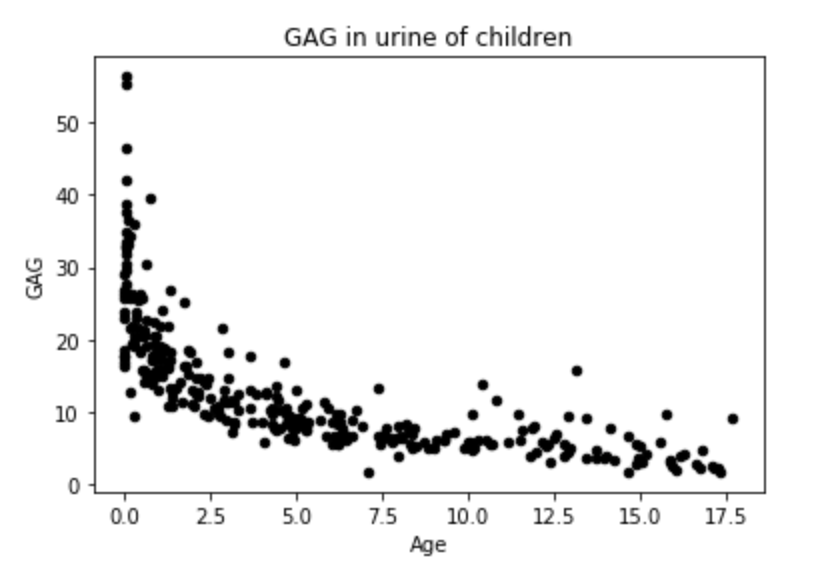Standard stuff: import function, convert variables to R format, call function

from rpy2.robjects.packages import importr
r_splines = importr('splines')# populate R variables
r_gag = robjects.FloatVector(GAGurine['GAG'].values)
r_age = robjects.FloatVector(GAGurine['Age'].values)
r_quarts = robjects.FloatVector(np.quantile(r_age,[.25,.5,.75])) #woah, numpy functions run on R objects


What happens when we call the ns or bs functions from r_splines?

ns_design = r_splines.ns(r_age, knots=r_quarts)
bsdesign = rsplines.bs(r_age, knots=r_quarts)
print(ns_design)


ns and bs return design matrices, not model objects! That's because they're meant to work with lm's formula interface. To get a model object we populate a formula including ns(<var>,<knots>) and fit to data.

r_lm = robjects.r['lm']
r_predict = robjects.r['predict']
populate the formula
ns_formula = robjects.Formula("Gag ~ ns(Age, knots=r_quarts)")
ns_formula.environment['Gag'] = r_gag
ns_formula.environment['Age'] = r_age
ns_formula.environment['r_quarts'] = r_quarts

fit the model
ns_model = r_lm(ns_formula


Predict like usual: build a dataframe to predict on and call predict() .

# predict
predict_frame = robjects.DataFrame({"Age": robjects.FloatVector(np.linspace(0,20,100))})ns_out = r_predict(ns_model, predict_frame)ax32 = GAGurine.plot.scatter(x='Age',y='GAG',c='grey',title="GAG in urine of children")
ax32.set_xlabel("Age")
ax32.set_ylabel("GAG")
ax32.plot(predict_frame.rx2("Age"),ns_out, color='red')
ax32.legend(["Natural spline, knots at quartiles"]);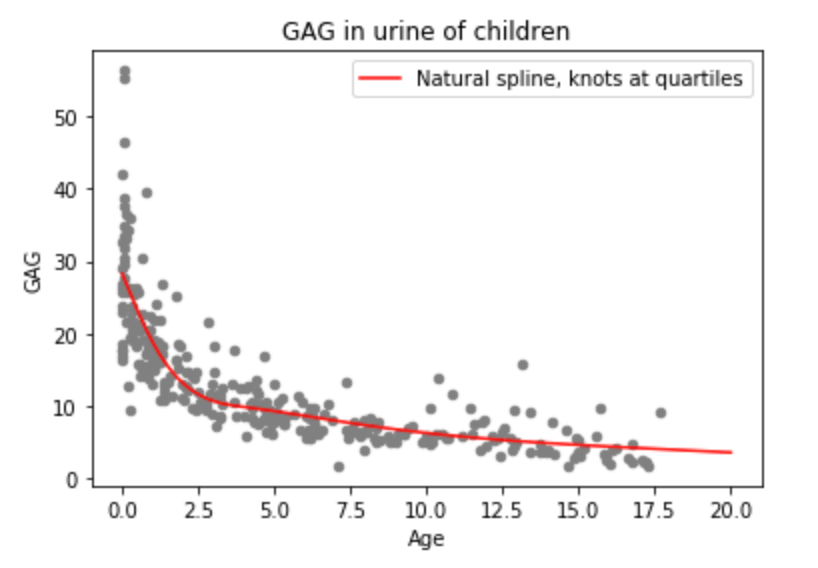Examples

Let’s look at two examples of implementing basis splines.

1. Fit a basis spline model with the same knots, and add it to the plot above.
bs_formula = robjects.Formula("Gag ~ bs(Age, knots=r_quarts)")
bs_formula.environment['Gag'] = r_gag
bs_formula.environment['Age'] = r_age
bs_formula.environment['r_quarts'] = r_quarts
bs_model = r_lm(bs_formula)
bs_out = r_predict(bs_model, predict_frame)ax32 = GAGurine.plot.scatter(x='Age',y='GAG',c='grey',title="GAG in urine of children")
ax32.set_xlabel("Age")
ax32.set_ylabel("GAG")
ax32.plot(predict_frame.rx2("Age"),ns_out, color='red')
ax32.plot(predict_frame.rx2("Age"),bs_out, color='blue')
ax32.legend(["Natural spline, knots at quartiles","B-spline, knots at quartiles"]);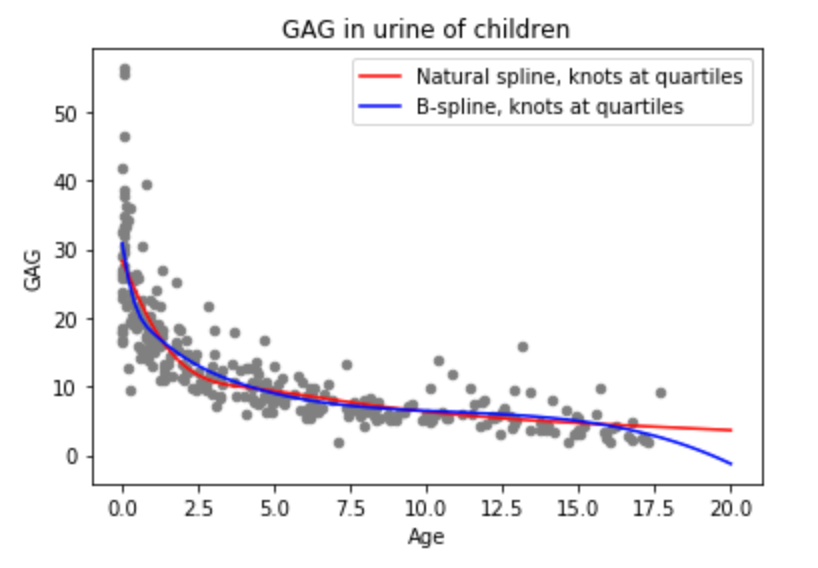2. Fit a basis spline with 8 knots placed at [2,4,6…14,16] and add it to the plot above.

overfit_formula = robjects.Formula("Gag ~ bs(Age, knots=r_quarts)")
overfit_formula.environment['Gag'] = r_gag
overfit_formula.environment['Age'] = r_age
overfit_formula.environment['r_quarts'] = robjects.FloatVector(np.array([2,4,6,8,10,12,14,16]))
overfit_model = r_lm(overfit_formula)
overfit_out = r_predict(overfit_model, predict_frame)ax32 = GAGurine.plot.scatter(x='Age',y='GAG',c='grey',title="GAG in urine of children")
ax32.set_xlabel("Age")
ax32.set_ylabel("GAG")
ax32.plot(predict_frame.rx2("Age"),ns_out, color='red')
ax32.plot(predict_frame.rx2("Age"),bs_out, color='blue')
ax32.plot(predict_frame.rx2("Age"),overfit_out, color='green')
ax32.legend(["Natural spline, knots at quartiles", "B-spline, knots at quartiles", "B-spline, lots of knots"]);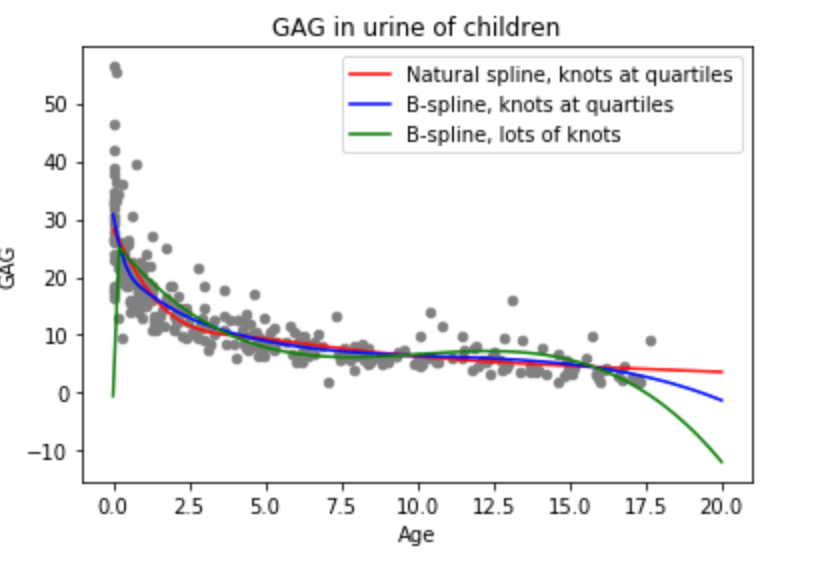GAMs

We come, at last, to our most advanced model. The coding here isn’t any more complex than we’ve done before, though the behind-the-scenes is awesome.

First, let’s get our multivariate data.

kyphosis = pd.read_csv("data/kyphosis.csv")print("""
kyphosis - wherther a particular deformation was present post-operation
age - patient's age in months
number - the number of vertebrae involved in the operation
start - the number of the topmost vertebrae operated on""")
display(kyphosis.describe(include='all'))
display(kyphosis.dtypes)#If there are errors about missing R packages, run the code below:
#r_utils = importr('utils')
#r_utils.install_packages('codetools')
#r_utils.install_packages('gam')


To fit a GAM, we

• Import the gam library
• Populate a formula including s(<var>) on variables which we want to smooth
• Call gam(formula, family=<string>) where family is a string naming a probability distribution, chosen based on how the response variable is thought to occur.

Rough family guidelines:

• Response is binary or “N occurrences out of M tries”, e.g. number of lab rats (out of 10) developing disease: choose "binomial"
• Response is a count with no logical upper bound, e.g. number of ice creams sold: choose "poisson"
• Response is real, with normally-distributed noise, e.g. person’s height: choose "gaussian" (the default)
#There is a Python library in development for using GAMs
(https://github.com/dswah/pyGAM)
but it is not yet as comprehensive as the R GAM library, which we will use here instead.
R also has the mgcv library, which implements some more advanced/flexible fitting methods
r_gam_lib = importr('gam')
r_gam = r_gam_lib.gam
r_kyph = robjects.FactorVector(kyphosis[["Kyphosis"]].values)
r_Age = robjects.FloatVector(kyphosis[["Age"]].values)
r_Number = robjects.FloatVector(kyphosis[["Number"]].values)
r_Start = robjects.FloatVector(kyphosis[["Start"]].values)
kyph1_fmla = robjects.Formula("Kyphosis ~ s(Age) + s(Number) + s(Start)")
kyph1_fmla.environment['Kyphosis']=r_kyph
kyph1_fmla.environment['Age']=r_Age
kyph1_fmla.environment['Number']=r_Number
kyph1_fmla.environment['Start']=r_Start
kyph1_gam = r_gam(kyph1_fmla, family="binomial")


The fitted gam model has a lot of interesting data within it:

print(kyph1_gam.names)


Remember plotting? Calling R’s plot() on a gam model is the easiest way to view the fitted splines

In [ ]:

%R -i kyph1_gam plot(kyph1_gam, residuals=TRUE,se=TRUE, scale=20);


Prediction works like normal (build a data frame to predict on, if you don’t already have one, and call predict()). However, predict always reports the sum of the individual variable effects. If family is non-default this can be different from the actual prediction for that point.

For instance, we’re doing a ‘logistic regression’ so the raw prediction is log-odds, but we can get probability by using in predict(..., type="response")

kyph_new = robjects.DataFrame({'Age': robjects.IntVector((84,85,86)),
'Start': robjects.IntVector((5,3,1)),
'Number': robjects.IntVector((1,6,10))})print("Raw response (so, Log odds):")
display(r_predict(kyph1_gam, kyph_new))
print("Scaled response (so, probabilty of kyphosis):")
display(r_predict(kyph1_gam, kyph_new, type="response"))


Using R functions in Python is relatively easy once you are familiar with the procedure, and it can save a lot of headaches if you need to use R packages to perform your data analysis or are a Python user who has been given R code to work with.

I hope you enjoyed this article and found it informative and useful. All the code used in this notebook can be found on my GitHub page for those of you who wish to experiment with interfacing between R and Python functions and objects in the Jupyter environment.

If you liked this post, share it with all of your programming buddies!

## Data Science Projects | Data Science | Machine Learning | Python

Practice your skills in Data Science with Python, by learning and then trying all these hands-on, interactive projects, that I have posted for you.

## Data Science Projects | Data Science | Machine Learning | Python

Practice your skills in Data Science with Python, by learning and then trying all these hands-on, interactive projects, that I have posted for you.

## Data Science Projects | Data Science | Machine Learning | Python

Practice your skills in Data Science with Python, by learning and then trying all these hands-on, interactive projects, that I have posted for you.

## Data Science Projects | Data Science | Machine Learning | Python

Practice your skills in Data Science with Python, by learning and then trying all these hands-on, interactive projects, that I have posted for you.

## Data Science Projects | Data Science | Machine Learning | Python

Practice your skills in Data Science with Python, by learning and then trying all these hands-on, interactive projects, that I have posted for you.